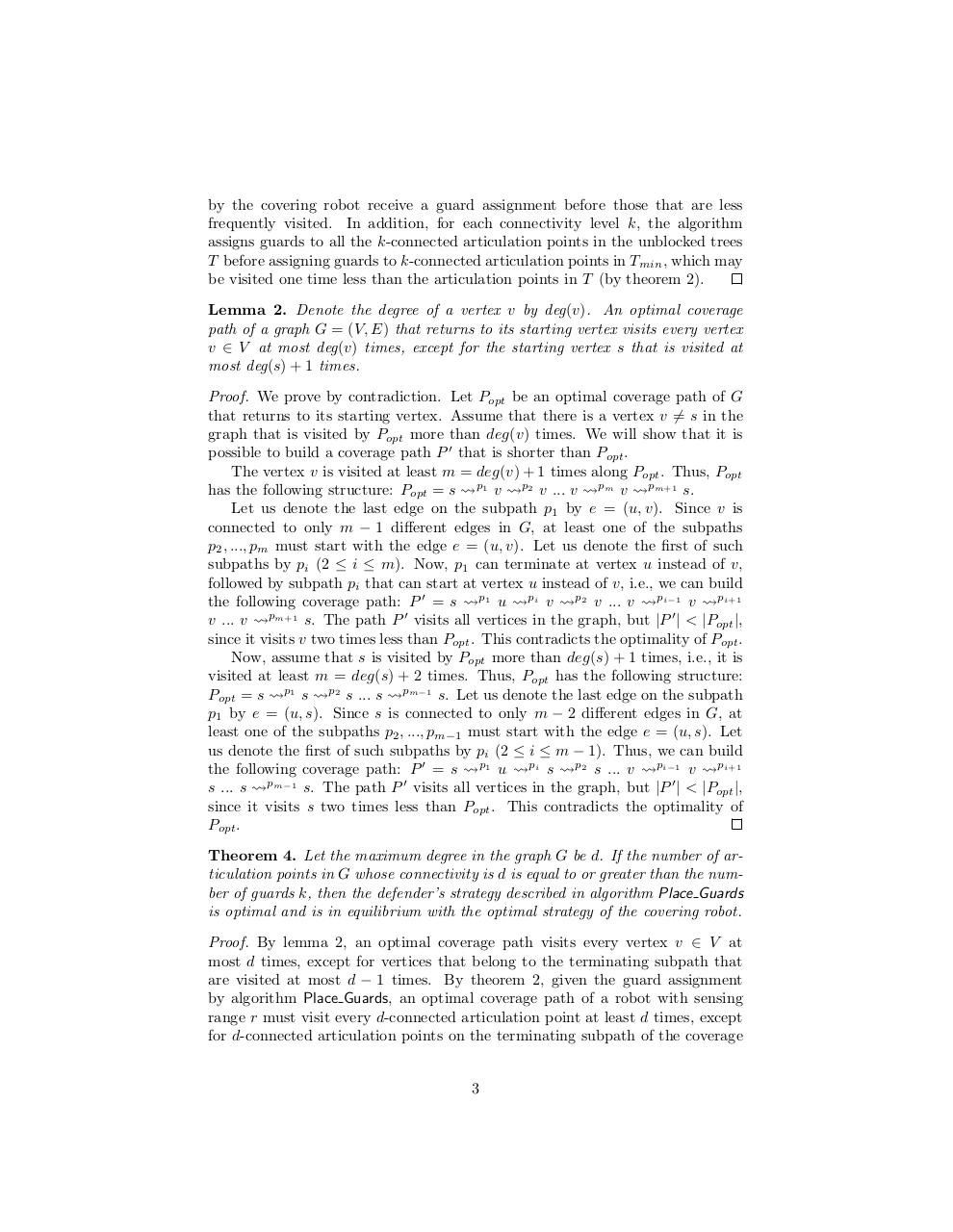Page 1 2 3 4 5 6 7 8 9 10

#### Text preview

by the covering robot receive a guard assignment before those that are less
frequently visited. In addition, for each connectivity level k, the algorithm
assigns guards to all the k-connected articulation points in the unblocked trees
T before assigning guards to k-connected articulation points in Tmin , which may
be visited one time less than the articulation points in T (by theorem 2).
Lemma 2. Denote the degree of a vertex v by deg(v). An optimal coverage
path of a graph G = (V, E) that returns to its starting vertex visits every vertex
v ∈ V at most deg(v) times, except for the starting vertex s that is visited at
most deg(s) + 1 times.
Proof. We prove by contradiction. Let Popt be an optimal coverage path of G
that returns to its starting vertex. Assume that there is a vertex v 6= s in the
graph that is visited by Popt more than deg(v) times. We will show that it is
possible to build a coverage path P 0 that is shorter than Popt .
The vertex v is visited at least m = deg(v) + 1 times along Popt . Thus, Popt
has the following structure: Popt = s p1 v p2 v ... v pm v pm+1 s.
Let us denote the last edge on the subpath p1 by e = (u, v). Since v is
connected to only m − 1 different edges in G, at least one of the subpaths
p2 , ..., pm must start with the edge e = (u, v). Let us denote the first of such
subpaths by pi (2 ≤ i ≤ m). Now, p1 can terminate at vertex u instead of v,
followed by subpath pi that can start at vertex u instead of v, i.e., we can build
the following coverage path: P 0 = s p1 u pi v p2 v ... v pi−1 v pi+1
v ... v pm+1 s. The path P 0 visits all vertices in the graph, but |P 0 | &lt; |Popt |,
since it visits v two times less than Popt . This contradicts the optimality of Popt .
Now, assume that s is visited by Popt more than deg(s) + 1 times, i.e., it is
visited at least m = deg(s) + 2 times. Thus, Popt has the following structure:
Popt = s p1 s p2 s ... s pm−1 s. Let us denote the last edge on the subpath
p1 by e = (u, s). Since s is connected to only m − 2 different edges in G, at
least one of the subpaths p2 , ..., pm−1 must start with the edge e = (u, s). Let
us denote the first of such subpaths by pi (2 ≤ i ≤ m − 1). Thus, we can build
the following coverage path: P 0 = s p1 u pi s p2 s ... v pi−1 v pi+1
s ... s pm−1 s. The path P 0 visits all vertices in the graph, but |P 0 | &lt; |Popt |,
since it visits s two times less than Popt . This contradicts the optimality of
Popt .
Theorem 4. Let the maximum degree in the graph G be d. If the number of articulation points in G whose connectivity is d is equal to or greater than the number of guards k, then the defender’s strategy described in algorithm Place Guards
is optimal and is in equilibrium with the optimal strategy of the covering robot.
Proof. By lemma 2, an optimal coverage path visits every vertex v ∈ V at
most d times, except for vertices that belong to the terminating subpath that
are visited at most d − 1 times. By theorem 2, given the guard assignment
by algorithm Place Guards, an optimal coverage path of a robot with sensing
range r must visit every d-connected articulation point at least d times, except
for d-connected articulation points on the terminating subpath of the coverage

3